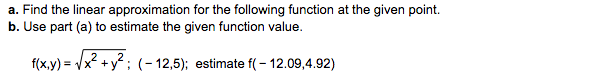# a. Find the linear approximation for the following function at the given pointb. Use part (a) to estimate the given function value.#xy)-A2+y2; (-12,5); estimatet(-1209,432)

Question
164 viewshelp_outlineImage Transcriptionclosea. Find the linear approximation for the following function at the given point b. Use part (a) to estimate the given function value. #xy)-A2+y2; (-12,5); estimatet(-1209,432) fullscreen
check_circle

Step 1

The formula for calculating linear approximation L(x,y) of f(x,y) is shown on board, so we calculate f(a,b), and the value of first order  partial derivatives at (a,b)

Step 2

Now, we substitute all the values to get expression for linear approximation.

And the...

### Want to see the full answer?

See Solution

#### Want to see this answer and more?

Solutions are written by subject experts who are available 24/7. Questions are typically answered within 1 hour.*

See Solution
*Response times may vary by subject and question.
Tagged in